#### The phase space

Unfortunately, motions are usually described in terms of accelerations (and sometimes higher-order derivatives), which need to be integrated twice. This leads to higher-order differential equations, which are difficult to work with. For this reason, phase space representations were developed in physics and engineering. In this case, the velocities of the state variables are themselves treated as state variables. That way, the accelerations become the velocities of the velocity variables.

For example, suppose that a position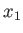is acted upon by gravity, which generates an acceleration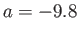m/s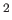. This leads to a second variable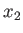, which is defined as the velocity of. Thus, by definition,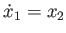. Furthermore,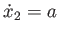because the derivative of velocity is acceleration. Both of these equations fit the form of (8.25). Generally, the number of states increases to incorporate accelerations (or even higher-order derivatives), but the resulting dynamics are expressed in the form (8.25), which is easier to work with.

Steven M LaValle 2020-11-11